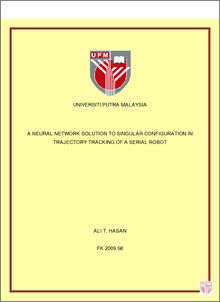Simple Search:

A Neural Network Solution to Singular Configuration in Trajectory Tracking of a Serial Robot

Citation

Hasan, Ali T. (2009) A Neural Network Solution to Singular Configuration in Trajectory Tracking of a Serial Robot. PhD thesis, Universiti Putra Malaysia.

Abstract / Synopsis

Singularities and uncertainties in arm configurations are the main problems in kinematics of serial robots. The complexity in the solution arises from robots geometry and non-linear equations (trigonometric equations) occur when transforming between Cartesian and joint spaces where multiple solutions and singularities exist. Mathematical solutions for the problem may not always correspond to the physical solution and methods of solution depend on the robot configuration. In this research, a trajectory tracking approach is proposed for a 6 Degrees Of Freedom (DOF) serial robot manipulator. The proposed solution is carried out through two stages. First the kinematics model of the Fanuc robot was solved using the D-H method to show the exact location of singular configurations of the robot, and then Artificial Neural Networks (ANNs) are trained to overcome these arising problems. Solving the Inverse Kinematics (IK) of serial manipulators by using ANNs has two problems, one of these is the selection of the appropriate configuration of the network and the other is the generating of suitable training data sets. In this research, although this is very difficult in practice, training data were recorded experimentally from sensors fixed on each joint to overcome the effect of kinematics uncertainties presence in the real world such as ill-defined linkage parameters, links flexibility and backlashes in gear train. Off-line training was implemented for the experimentally obtained training data. Two networks configurations from the literature were tested and developed following the recommendations of the original authors, then compared to find the best configuration to be used. First the effect of orientation of the tool was examined (as one of the networks does not considered the effect of orientation while the other network does), and then the effect of the Jacobian matrix to the solution for the both configurations was examined. Performance comparison shows that when the effect of the orientation of the tool was considered in the solution with the Jacobian matrix effect, better results in terms of precision and iteration during training the ANN were obtained. The effect of the network architecture was also examined in order to find the best network configuration to solve the problem. A network with all the parameters considered together in one network has been compared to six different networks, where the parameters of every joint were considered independently. Results obtained show that having one network considering all the problem’s parameters together give a better response than using 6 different networks representing the parameters of each joint apart from other joints. The resultant network with the best configuration was tested experimentally using new different set of data that has never been introduced to the network before, this data set was meant to pass through the singular configurations, in order to show the generality and efficiency of the proposed approach. Experimental trajectory tracking has shown the ability of the proposed Artificial Neural Networks approach to overcome the disadvantages of using some schemes like the Fuzzy Learning Control for example that only remembers the most recent data sets introduced, as the literature has shown.Preview
PDF
ABS_====__FK_2009_56.pdfView Item